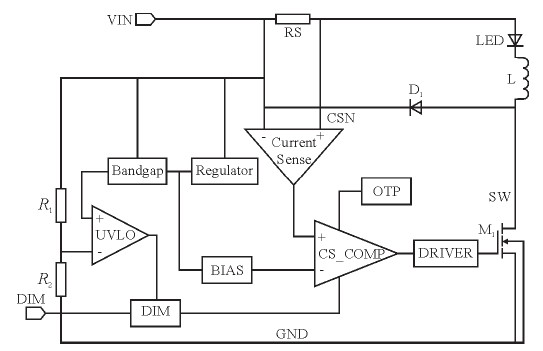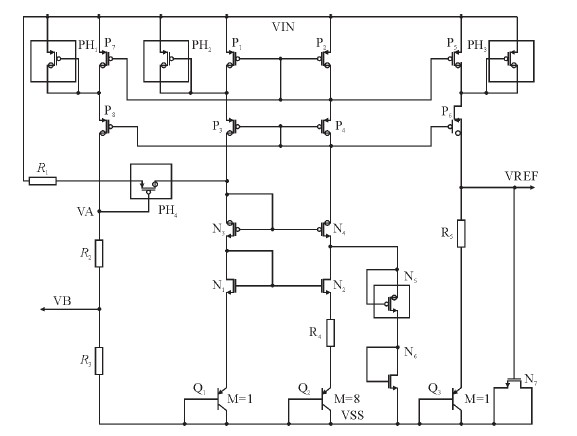# High efficiency, high dimming ratio LED constant current drive circuit design

introduction

With the development of LED technology, high-power LEDs have been widely used in lighting decoration and lighting, and power LED driver chips are becoming more and more important. Since the brightness output of the LED is proportional to the current through the LED, in order to ensure the brightness and chromaticity of each LED, it is necessary to design a constant current driver to make the LED current as uniform as possible.

Based on the LED illumination characteristics, this paper designs a wide voltage input, high current, high dimming ratio LED constant current driver chip. The chip uses a hysteresis current control mode that can be used to drive one or more LEDs in series. In the wide input voltage range of 6V~30V, the average current of the LED is set by sampling the high-side current, and the chip output current precision is controlled at 5.5%. At the same time, the chip can realize analog dimming and PWM dimming through the DIM pin. The chip's response speed allows the chip to achieve a high dimming ratio.

This paper first analyzes the overall circuit, then introduces the design of each important sub-module, and finally gives the overall simulation waveform, layout and conclusion of the chip.

1 circuit system principle

The circuit includes a bandgap reference, voltage regulator, high-side current sampling, hysteresis comparator, power transistor M1, PWM, and analog dimming. In addition, the chip also has built-in undervoltage and overtemperature protection circuits, which can effectively ensure the system can work stably under various adverse conditions.Figure 1 chip overall equivalent architecture

It can be seen from Figure 1 that the inductor L, the current sampling resistor RS, and the freewheeling diode D1 form a self-oscillating continuous inductor current mode constant current LED controller. The chip uses hysteresis current control mode, because the change of LED drive current reflects the change of voltage difference across RS, so when the circuit works normally, the current in the LED is sampled by sampling resistor RS and converted into a certain proportion of sampling. The voltage VCS, then the VCS enters the hysteresis comparator, and compares with the bias voltage generated by the BIAS module to generate a PWM control signal, and then controls the turn-on and turn-off of the power switch tube via the gate drive circuit.

The following is a detailed analysis of the working principle of the circuit. First, the chip is designed with two current thresholds IMAX and IMIN. When the power supply VIN is powered up, the initial current of the inductor L and the current sampling resistor RS is zero, and the LED current is also zero. At this time, the output of the CS_COMP hysteresis comparator is high, the built-in power NMOS switch M1 is turned on, the potential of the SW terminal is low, and the current flowing through the LED starts to rise. The current flows from VIN to ground through inductor L, current sense resistor RS, LED, and internal power switch. The slope of current rise is determined by VIN, inductor (L), and LED drop. When the LED current increases to the preset value IMAX, the output of the CS_COMP hysteresis comparator is low, and the power switch tube M1 is turned off. Due to the continuity of the inductor current, the current flows through the inductor (L) with another falling slope. Current sampling resistor (RS), LED and freewheeling Schottky diode (D1), when the current drops to another predetermined value IMIN, the power switch is turned back on, the power supply charges the inductor L, and the LED current begins to increase again. When the current increases to IMAX, the control circuit turns off the power transistor and repeats the last cycle of operation, thus completing the hysteresis control of the LED current, so that the average current of the LED is constant.

From the above analysis, the average driving current of the LED is determined by the built-in thresholds IMAX and IMIN, so there is no feedback loop similar to the peak current control mode. Therefore, compared with the peak current control mode, the hysteresis current control mode has self-stability and does not require a compensation circuit. In addition, the peak current detection mode dynamic response adjustment generally takes several cycles, while the hysteresis current control can take up to one cycle. Stabilizing the dynamic response of the system, so the dynamic response of hysteresis current control is more rapid. Of course, hysteresis current control mode has the disadvantages of large output ripple and variable frequency control, so in the high-power LED lighting drive application, certain ripple changes and switching frequency changes will not affect the overall illumination performance of the LED. Have a big impact.

2 circuit sub-module design

2.1 Bandgap reference (Bandgap)

Figure 2 shows a CMOS self-biased reference circuit with cascode current mirrors that improves power supply rejection and initial accuracy. Among them, R1 and PH4 form a starting circuit. When the power is turned on, if the circuit has a zero current state, VA is low, MOS tube PH4 is turned on, and current is injected into the reference core circuit, so that the reference circuit is freed from zero degeneracy. Set point, when the circuit works normally, make them all in the deep linear region by reasonably setting the width to length ratio of P7 and P8. Since the resistance values â€‹â€‹of R2 and R3 are large, the size of VA is close to the input voltage, MOS tube PH4 Shutdown, the startup ends. In addition, since the voltage of the VA is close to the power supply voltage, after the voltage division of the resistors R2 and R3, the voltage VB can characterize the power supply voltage, so that when the power supply voltage is lower than the set value, the undervoltage signal is output, and the power tube is turned off. The function to undervoltage protection.Figure 2 Bandgap reference voltage source circuit diagram

Since the input voltage of the reference circuit can reach up to 30V, the common MOS tube drain source and gate withstand voltage are 5V. And in order to make the current mirror more matching, P1, P2, P5, P7 must use ordinary MOS tubes. Therefore, in order to prevent the tube from being broken down at high voltage, it is necessary to add a gate-drained thick gate oxide MOS tube as a protection tube between the drain and the source of the tubes, that is, PH1, PH2, and PH3.